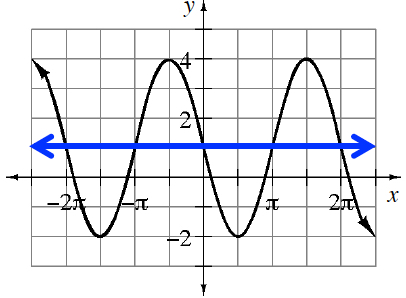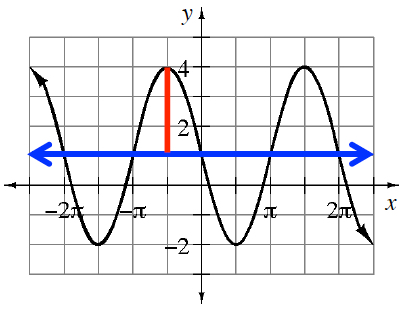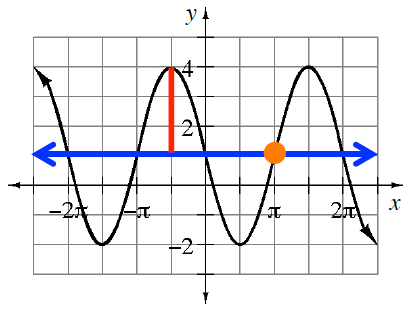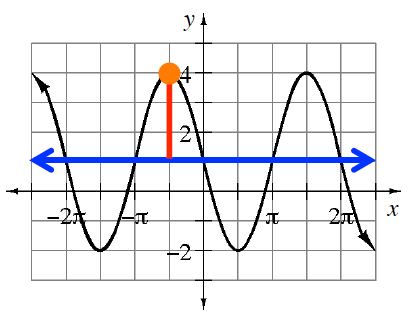### Home > PC3 > Chapter 3 > Lesson 3.1.5 > Problem3-82

3-82.

Write one equation in terms of sine and one equation in terms of cosine for the graph shown at right.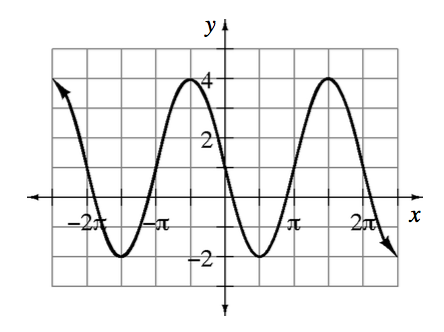Where is the midline of the curve? This is the vertical shift of the function.

What is the amplitude?

What is the simplest horizontal shift for the sine?

What is the simplest horizontal shift for the cosine?